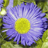# knowing the field name of calculated field in Summary Statistics using model builder

3214
5
03-09-2012 09:30 AMNew Contributor III
Hi,

I am running summary statistics in model builder on a table in which the statistics field is a parameter entered by the user (I am using the MAX function on this field). I then need to join the output of summary statistics to the input table (using the calculated statistic as the join field), and then use Copy Rows to export the join to a new table.

How do I know what the join field name is for the output of summary statistics?

Edit: I am using 9.3.1 if that matters.
Tags (3)
1 Solution

Accepted SolutionsbyMVP Esteemed Contributor

How do I know what the output statistic field name is for the output of summary statistics?

as the join field name should not change with different statistics, it would be the same as the case field.

The Calculate Value tool is the way to calculate the output statistic name, you enter a python expression to calculate the field name, and set the output data type to Field.  An example expression would be:

"%Statistic%_%Input field%"

where Statistic and Input field are elements in the model.
5 RepliesbyMVP Esteemed Contributor

How do I know what the output statistic field name is for the output of summary statistics?

as the join field name should not change with different statistics, it would be the same as the case field.

The Calculate Value tool is the way to calculate the output statistic name, you enter a python expression to calculate the field name, and set the output data type to Field.  An example expression would be:

"%Statistic%_%Input field%"

where Statistic and Input field are elements in the model.New Contributor III
Thanks for the reply. It appears you must have Spatial Analyst or 3D Analyst installed to use Calculate Value. I need to pass this custom tool on eventually and I want to make as few assumptions on their environment as possible. Is there any other way to do this?New Contributor III
Perhaps I didn't understand the requirement correctly and you only need Spatial or 3D Analyst if you have an ArcView or ArcEditor license. It's safe for me to assume they have an ArcInfo license.

Calculate Value worked. An added requirement for anyone trying this in the future, remember to set the output table of Summary Statistics as a precondition for the Calculate Value tool.byMVP Esteemed Contributor
Perhaps I didn't understand the requirement correctly and you only need Spatial or 3D Analyst if you have an ArcView or ArcEditor license. It's safe for me to assume they have an ArcInfo license.

Calculate Value worked. An added requirement for anyone trying this in the future, remember to set the output table of Summary Statistics as a precondition for the Calculate Value tool.

Great!

It sure would be great if Calculate Value didn't require advanced licensing - it's awfully hard to make a desktop tool using ModelBuilder without it.

Models will show a different icon and won't run if the any of the license/installs needed for its components are not available. I have found when building tools of any significant complexity that the effort of creating a python script is worth it - as then you can do things like more sophisticated tool parameter validation code and messages. (A nice improvement of 10.0 is that you can import scripts into the toolbox so you don't need to package a folder of scripts with the toolbox.)byMVP Esteemed Contributor

UPDATE as of now (since 10.0 at least) Calculate Value does not require advanced licensing, it works with all license levels.